# RS Aggarwal Solutions for Class 7 Maths Exercise 20A Chapter 20 Mensuration

RS Aggarwal Solutions for Class 7 Maths Exercise 20A Chapter 20 Mensuration in simple PDF are given here. This exercise of RS Aggarwal Solutions for Class 7 Chapter 20 contains questions related to areas of rectilinear figures, closed figures, simple figures, area and perimeter of rectangle and square. A figure made up of some line segments is called a rectilinear figure. Students can learn more about these topics by solving the questions of RS Aggarwal Solutions for Class 7 Maths Chapter 20 Mensuration with the help of solutions provided here.

## Download the PDF of RS Aggarwal Solutions For Class 7 Maths Chapter 20 Mensuration – Exercise 20A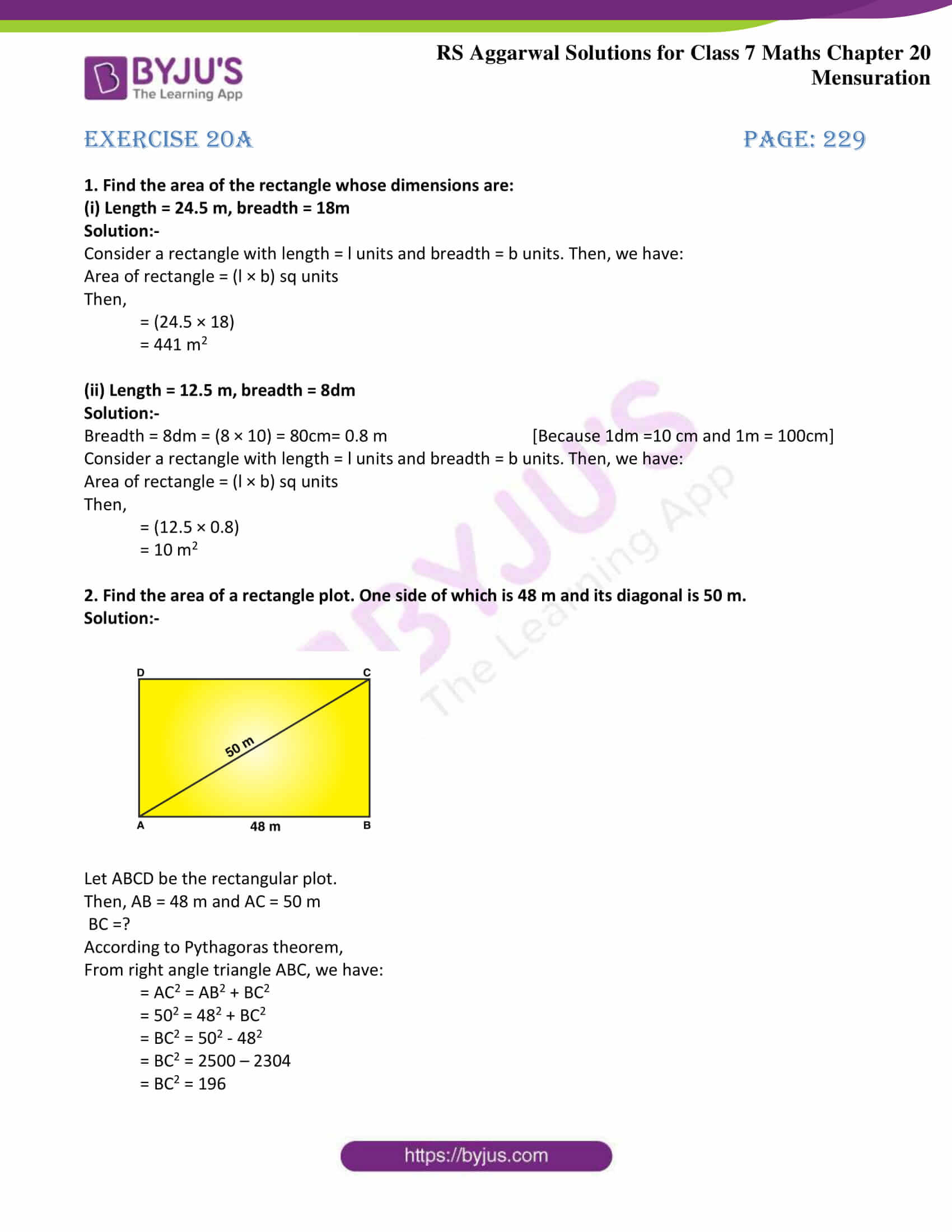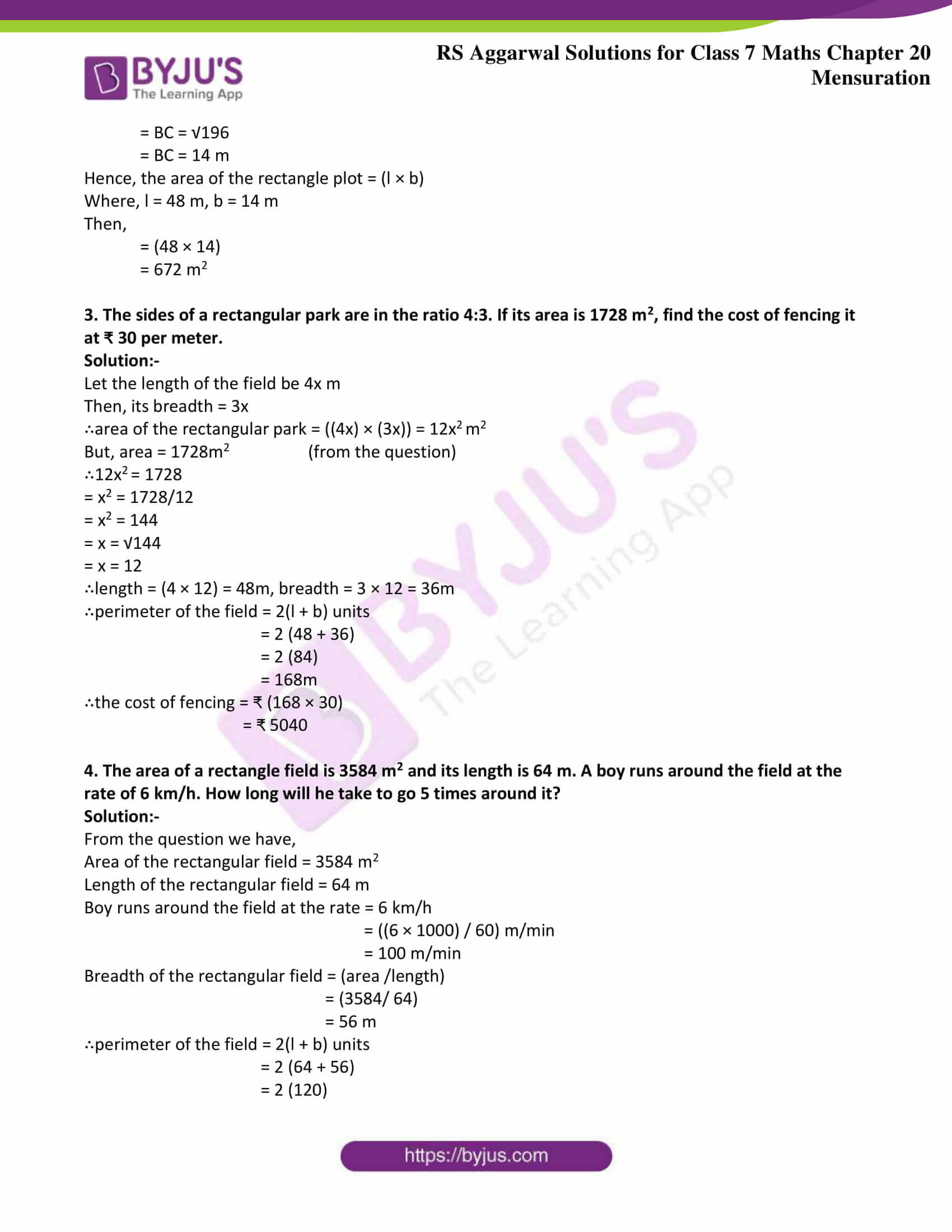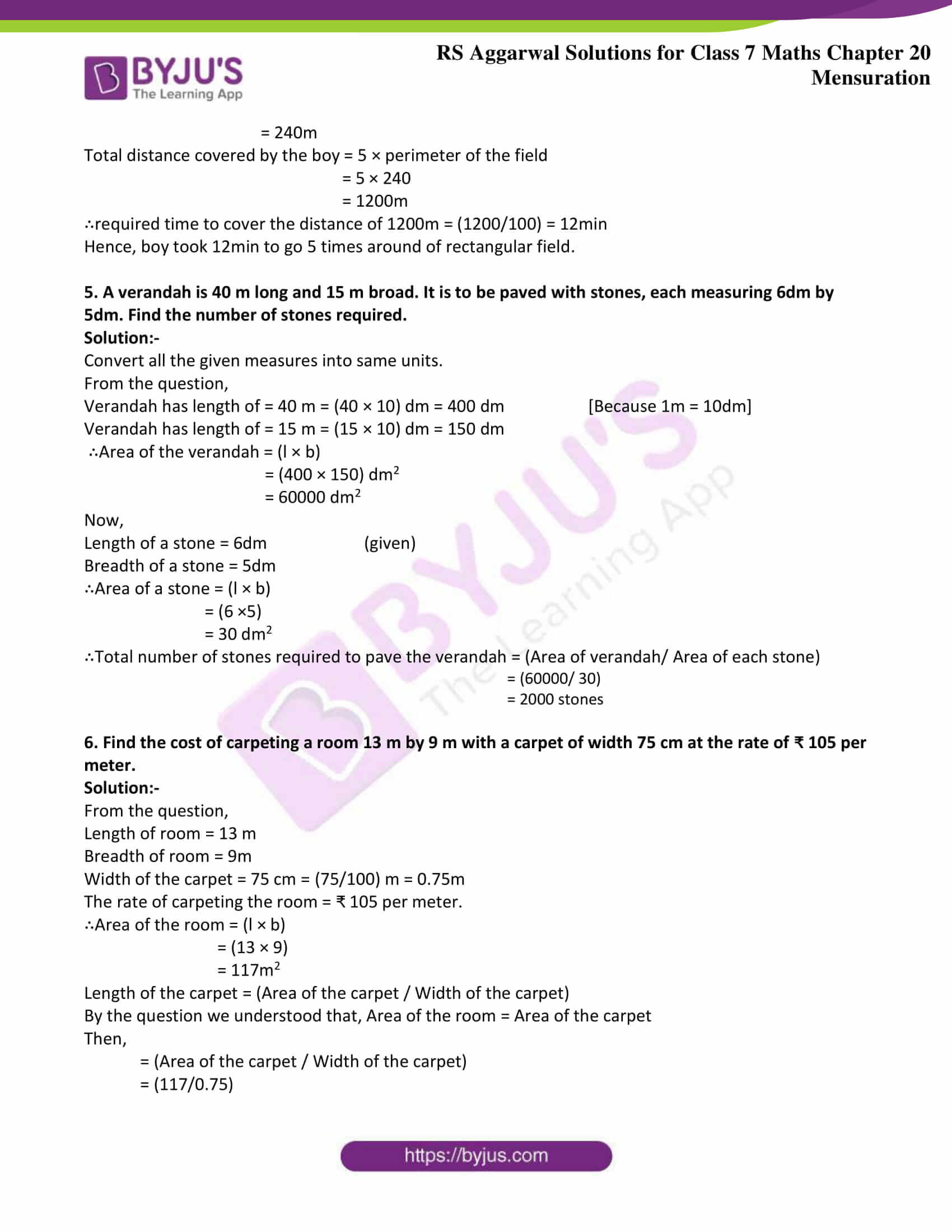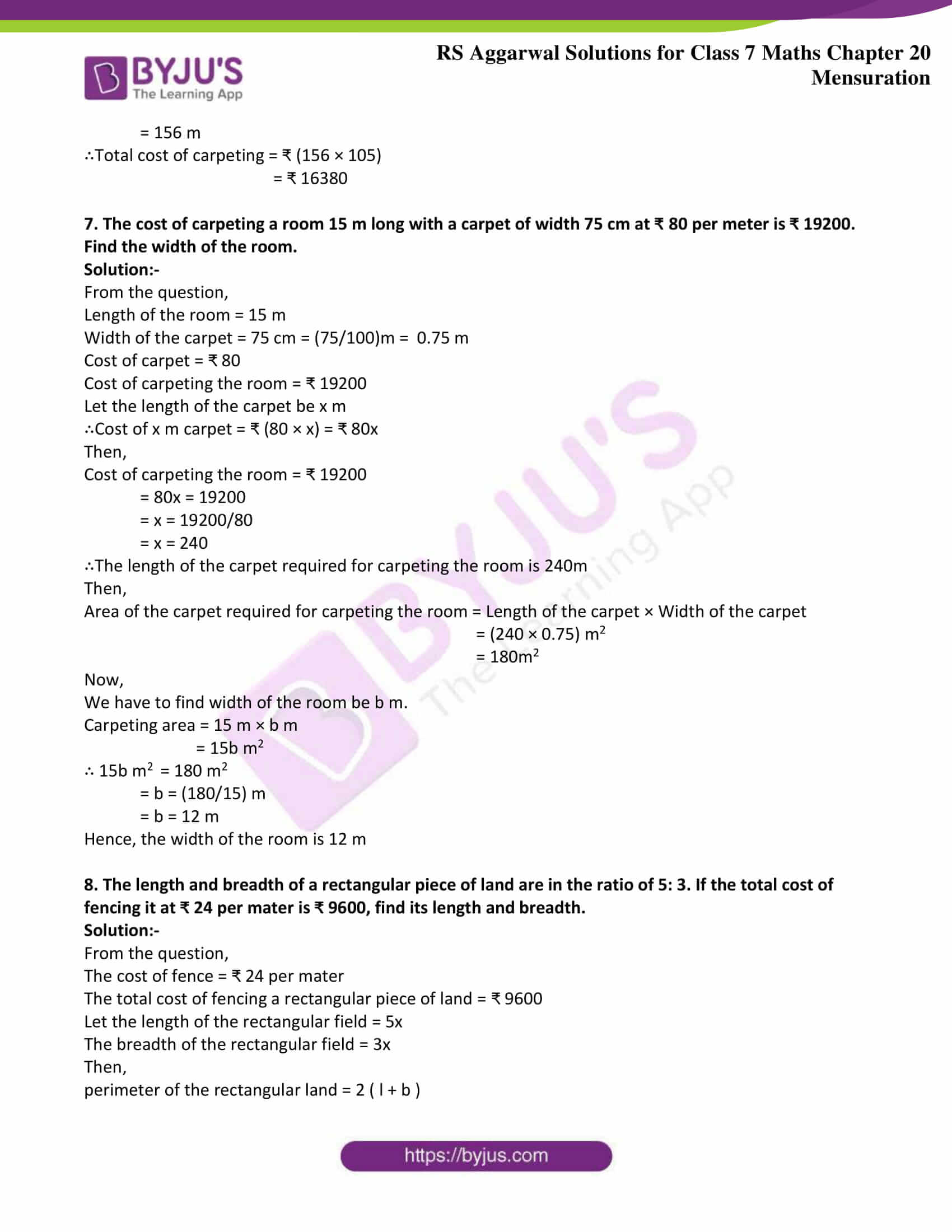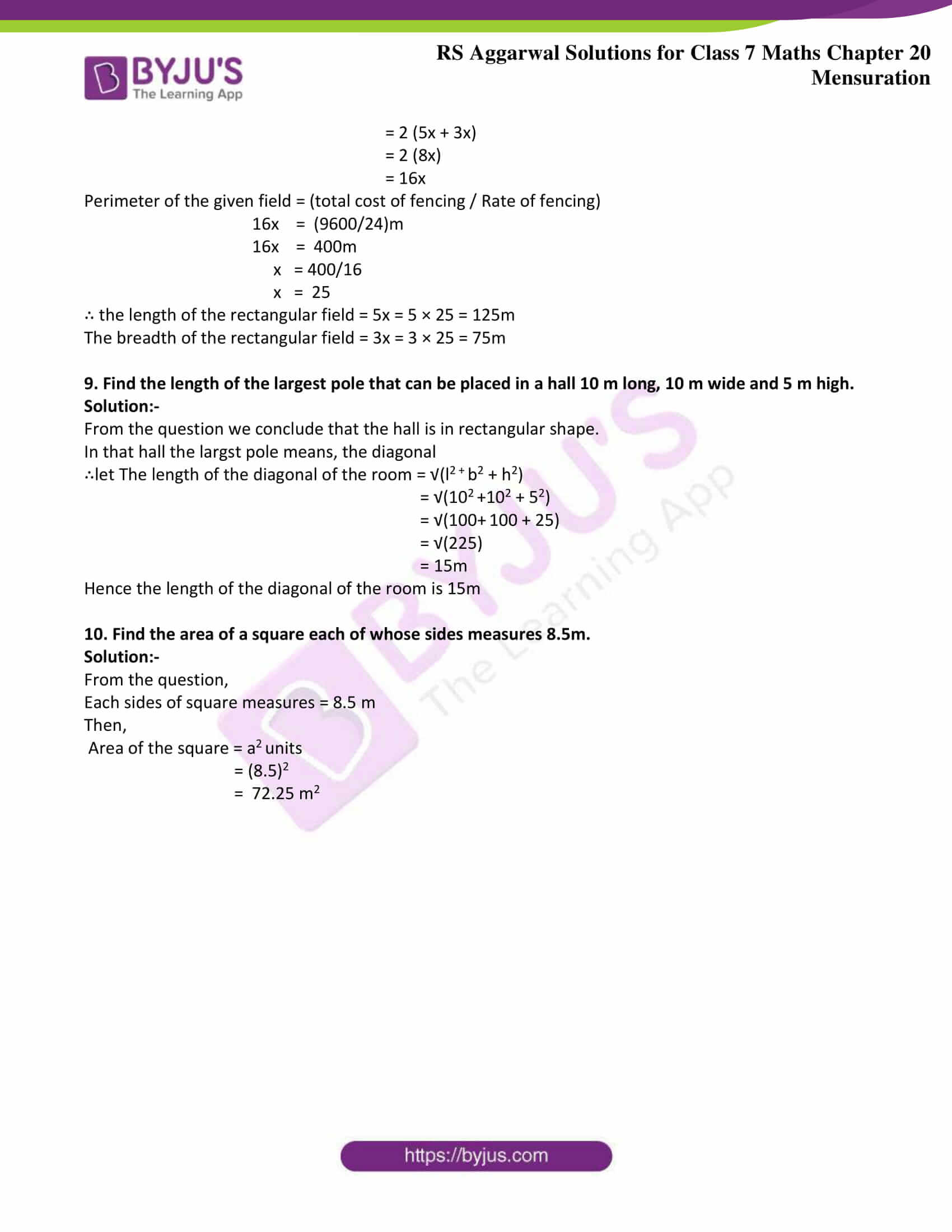### Access answers to Maths RS Aggarwal Solutions for Class 7 Chapter 20 – Mensuration Exercise 20A

1. Find the area of the rectangle whose dimensions are:

(i) Length = 24.5 m, breadth = 18m

Solution:-

Consider a rectangle with length = l units and breadth = b units. Then, we have:

Area of rectangle = (l × b) sq units

Then,

= (24.5 × 18)

= 441 m2

(ii) Length = 12.5 m, breadth = 8dm

Solution:-

Breadth = 8dm = (8 × 10) = 80cm= 0.8 m [Because 1dm =10 cm and 1m = 100cm]

Consider a rectangle with length = l units and breadth = b units. Then, we have:

Area of rectangle = (l × b) sq units

Then,

= (12.5 × 0.8)

= 10 m2

2. Find the area of a rectangle plot. One side of which is 48 m and its diagonal is 50 m.

Solution:-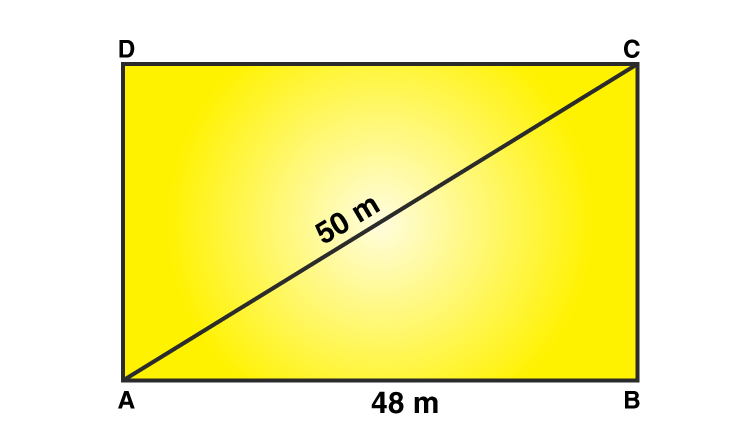Let ABCD be the rectangular plot.

Then, AB = 48 m and AC = 50 m

BC =?

According to Pythagoras theorem,

From right angle triangle ABC, we have:

= AC2 = AB2 + BC2

= 502 = 482 + BC2

= BC2 = 502 – 482

= BC2 = 2500 – 2304

= BC2 = 196

= BC = √196

= BC = 14 m

Hence, the area of the rectangle plot = (l × b)

Where, l = 48 m, b = 14 m

Then,

= (48 × 14)

= 672 m2

3. The sides of a rectangular park are in the ratio 4:3. If its area is 1728 m2, find the cost of fencing it at ₹ 30 per meter.

Solution:-

Let the length of the field be 4x m

∴area of the rectangular park = ((4x) × (3x)) = 12x2 m2

But, area = 1728m2 (from the question)

∴12x2 = 1728

= x2 = 1728/12

= x2 = 144

= x = √144

= x = 12

∴length = (4 × 12) = 48m, breadth = 3 × 12 = 36m

∴perimeter of the field = 2(l + b) units

= 2 (48 + 36)

= 2 (84)

= 168m

∴the cost of fencing = ₹ (168 × 30)

= ₹ 5040

4. The area of a rectangle field is 3584 m2 and its length is 64 m. A boy runs around the field at the rate of 6 km/h. How long will he take to go 5 times around it?

Solution:-

From the question we have,

Area of the rectangular field = 3584 m2

Length of the rectangular field = 64 m

Boy runs around the field at the rate = 6 km/h

= ((6 × 1000) / 60) m/min

= 100 m/min

Breadth of the rectangular field = (area /length)

= (3584/ 64)

= 56 m

∴perimeter of the field = 2(l + b) units

= 2 (64 + 56)

= 2 (120)

= 240m

Total distance covered by the boy = 5 × perimeter of the field

= 5 × 240

= 1200m

∴required time to cover the distance of 1200m = (1200/100) = 12min

Hence, boy took 12min to go 5 times around of rectangular field.

5. A verandah is 40 m long and 15 m broad. It is to be paved with stones, each measuring 6dm by 5dm. Find the number of stones required.

Solution:-

Convert all the given measures into same units.

From the question,

Verandah has length of = 40 m = (40 × 10) dm = 400 dm [Because 1m = 10dm]

Verandah has length of = 15 m = (15 × 10) dm = 150 dm

∴Area of the verandah = (l × b)

= (400 × 150) dm2

= 60000 dm2

Now,

Length of a stone = 6dm (given)

Breadth of a stone = 5dm

∴Area of a stone = (l × b)

= (6 ×5)

= 30 dm2

∴Total number of stones required to pave the verandah = (Area of verandah/ Area of each stone)

= (60000/ 30)

= 2000 stones

6. Find the cost of carpeting a room 13 m by 9 m with a carpet of width 75 cm at the rate of ₹ 105 per meter.

Solution:-

From the question,

Length of room = 13 m

Width of the carpet = 75 cm = (75/100) m = 0.75m

The rate of carpeting the room = ₹ 105 per meter.

∴Area of the room = (l × b)

= (13 × 9)

= 117m2

Length of the carpet = (Area of the carpet / Width of the carpet)

By the question we understood that, Area of the room = Area of the carpet

Then,

= (Area of the carpet / Width of the carpet)

= (117/0.75)

= 156 m

∴Total cost of carpeting = ₹ (156 × 105)

= ₹ 16380

7. The cost of carpeting a room 15 m long with a carpet of width 75 cm at ₹ 80 per meter is ₹ 19200. Find the width of the room.

Solution:-

From the question,

Length of the room = 15 m

Width of the carpet = 75 cm = (75/100)m = 0.75 m

Cost of carpet = ₹ 80

Cost of carpeting the room = ₹ 19200

Let the length of the carpet be x m

∴Cost of x m carpet = ₹ (80 × x) = ₹ 80x

Then,

Cost of carpeting the room = ₹ 19200

= 80x = 19200

= x = 19200/80

= x = 240

∴The length of the carpet required for carpeting the room is 240m

Then,

Area of the carpet required for carpeting the room = Length of the carpet × Width of the carpet

= (240 × 0.75) m2

= 180m2

Now,

We have to find width of the room be b m.

Carpeting area = 15 m × b m

= 15b m2

∴ 15b m2 = 180 m2

= b = (180/15) m

= b = 12 m

Hence, the width of the room is 12 m

8. The length and breadth of a rectangular piece of land are in the ratio of 5: 3. If the total cost of fencing it at ₹ 24 per mater is ₹ 9600, find its length and breadth.

Solution:-

From the question,

The cost of fence = ₹ 24 per mater

The total cost of fencing a rectangular piece of land = ₹ 9600

Let the length of the rectangular field = 5x

The breadth of the rectangular field = 3x

Then,

perimeter of the rectangular land = 2 ( l + b )

= 2 (5x + 3x)

= 2 (8x)

= 16x

Perimeter of the given field = (total cost of fencing / Rate of fencing)

16x = (9600/24)m

16x = 400m

x = 400/16

x = 25

∴ the length of the rectangular field = 5x = 5 × 25 = 125m

The breadth of the rectangular field = 3x = 3 × 25 = 75m

9. Find the length of the largest pole that can be placed in a hall 10 m long, 10 m wide and 5 m high.

Solution:-

From the question we conclude that the hall is in rectangular shape.

In that hall the largst pole means, the diagonal

∴let The length of the diagonal of the room = √(l2 + b2 + h2)

= √(102 +102 + 52)

= √(100+ 100 + 25)

= √(225)

= 15m

Hence the length of the diagonal of the room is 15m

10. Find the area of a square each of whose sides measures 8.5m.

Solution:-

From the question,

Each sides of square measures = 8.5 m

Then,

Area of the square = a2 units

= (8.5)2

= 72.25 m2

### Access other exercises of RS Aggarwal Solutions For Class 7 Chapter 20 – Mensuration

Exercise 20B Solutions

Exercise 20C Solutions

Exercise 20D Solutions

Exercise 20E Solutions

Exercise 20F Solutions

Exercise 20G Solutions